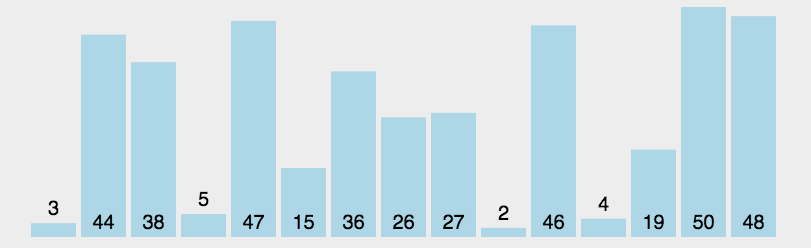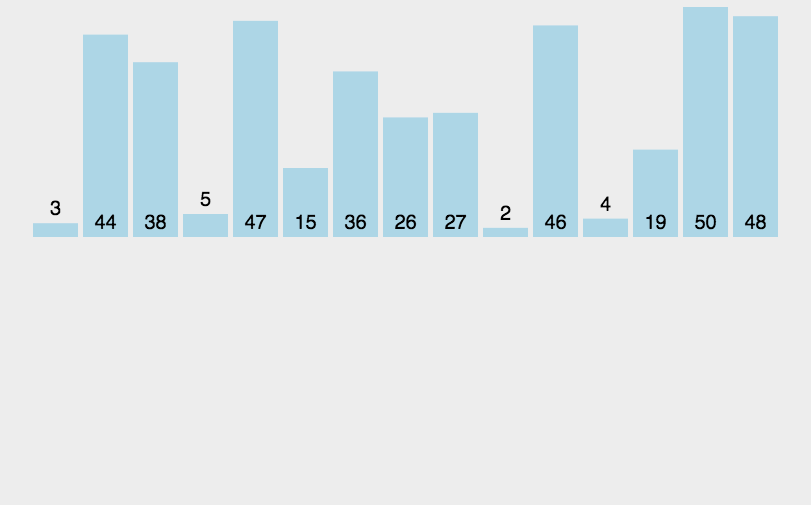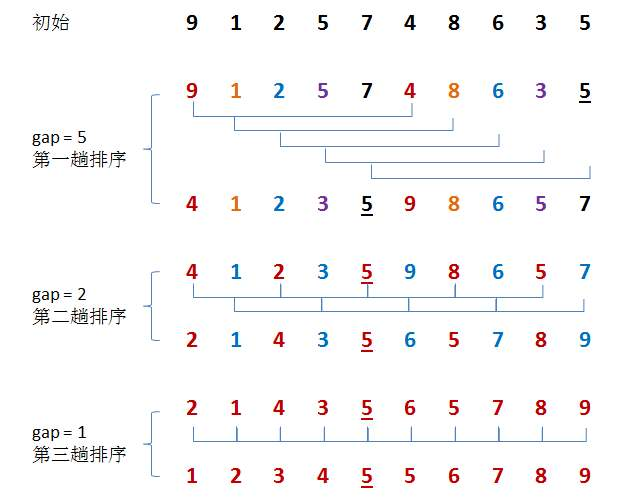ps.没有讲到稳定性和空间复杂度。

• 时间复杂度大约为O(n^2),可进行一些优化
这应该是c语言课程里头第一次讲到的排序算法，可以说得上是所有排序算法里头最简单的算法了。
• 思想：按一定的顺序，比如要求从大到小进行排序，那么第一位到最后一位（也可从最后一位到第一位）依次进行多次比较
• 这个算法的名字由来是因为越小的元素会经由交换慢慢“浮”到数列的顶端（升序或降序排列），就如同碳酸饮料中二氧化碳的气泡最终会上浮到顶端一样，故名“冒泡排序”。

 1//这里按从小到大排序 2for(int i=0;i<n;i++)//比较n轮 3for(int j=0;j<n,j++)//n轮中每一轮比较n次 4{ 5  if(a[j]>a[j+1]) 6  { 7    temp=a[j]; 8    a[j]=a[j+1]; 9    a[j+1]=temp;10  }11} 

 1for(int i=0;i<n;i++)//比较n轮 2for(int j=0;j<n-i;j++)//每一轮比较n-j次 3{ 4  if(a[j]>a[j+1]) 5  { 6    temp=a[j]; 7    a[j]=a[j+1]; 8    a[j+1]=temp; 9  }10}

02.直接选择排序

• ps.其实并不一定是从小到大，也可从大到小。 选择排序的时间复杂度也是O(n^2)

• 思想：也是和冒泡一样，进行多轮比较，但不一样的地方在于，经过每一次比较之后，每一轮会确定一个最小（大）的数对应的位置，最后才进行一次交换，相比之下，冒泡是一直在交换，事实上，冒泡和直接选择排序一样，比较低端，能不用尽量不用，尤其对长度较大的数组。

代码实现

 1content_copy 2int temp; 3for(int i=0;i<n-1;i++) 4{ 5  int k=i; 6  for(int j=i+1;j<n;j++) 7    if(a[j]<a[k]) 8    k=j;//每一轮中选出最小的数组元素对应的下标 9temp=a[k];10a[k]=a[i];11a[i]=temp;12}在这里插入图片描述
03.直接插入排序

Sort）是一种最简单的排序方法，其基本操作是将一条记录插入到已排好的有序表中，从而得到一个新的、记录数量增1的有序表。

• 直接插入排序的时间复杂度还是O(n^2)
• 思想：每次选择一个元素，将这个元素与数组中该元素之前所有的元素进行比较，然后将它插到合适的位置。

1    int i,j,temp;2    for ( i = 1; i < n; i++) {3         temp = a[i];//每一轮选出一个元素4        for ( j = i; j > 0 && a[j - 1] > temp; j--) {5            a[j] = a[j - 1];//与前面的元素比较大小，然后插进去，后面的元素退一位6        }7        a[j] = temp;8    }在这里插入图片描述
04.希尔排序

Sort），是直接插入排序算法的一种更高效的改进版本。希尔排序是非稳定排序算法。该方法因D.L.Shell于1959年提出而得名。

• 在某些极端情况下，希尔排序的时间复杂度会达到O(n^2)

• 希尔排序的平均时间复杂度是O(n^(1.3—2))
思想：直接插入排序要进行多次的比较交换，如果说，一个数组中大部分元素都处于有序的状态下，那么就不需要进行多次的比较和交换了。那么就需要先对数组进行一定的处理。

• 以下是希尔增量下的希尔排序，关于希尔排序的增量，有很多种选择，例如Hibbard增量，这些增量有些是通过数学证明得到的，有些则是还没有得到证明的，人们确信正确的经验得出的。。个人感觉有点像孪生素数猜想那样的吧，但证明难度应该没有那么高。

 1  for (int gap= n/2; gap > 0; gap /= 2)//分组 2    { 3        for (int i = gap; i < n; i ++ ) 4        { 5            int temp = a[i]; 6            int j;          //这里基本上和插入排序差不多 7            for (j = i; j >= gap && a[j - gap] > temp; j -= gap) 8                a[j] = a[j - gap]; 9            a[j] = temp;10        }11    }

• ps.希尔排序的动图我在网上找不到，只能用图片代替了
对于这样的一个数组，进行分组，gap=n/2，然后每分好之后，再gap/=2，一直到gap=1，这个过程使得数组的整体有序性提高，从而使直接插入排序的工作量减少很多.在这里插入图片描述
对于其它增量实现的，这里不贴出来了，因为，我不会写唉(逃

posted @ 2019-12-20 15:44  answerer  阅读(...)  评论(...编辑  收藏